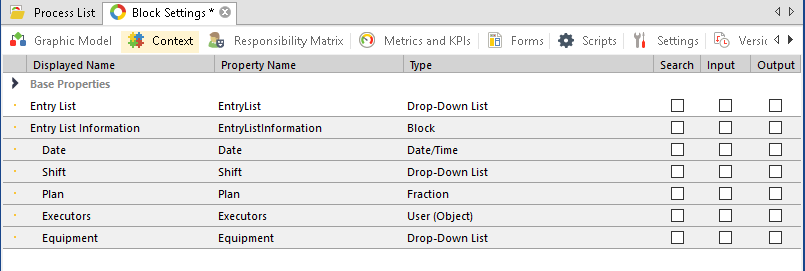# Moving data from an Excel file to a block

This example describes getting data from an *.xlsx file and writing them to a block using a script.
As an example, we will use a file with average grades of students. Add a block to the process context with fields Last Name (String type), Average Grade (Fraction type) and File variable (File type).
For the script to work, add the assembly:
Aspose.Cells
Namespaces:
``````using Aspose.Cells;
using EleWise.ELMA.Model.Common;
using EleWise.ELMA.Model.Entities;
using EleWise.ELMA.Model.Entities.ProcessContext;
using EleWise.ELMA.Model.Managers;
using EleWise.ELMA.Model.Types.Settings;
using EleWise.ELMA.Model.Services;``````

Script text:
``````int StartRow=1; //row number in the Excel file, to start writing Date with (numeration starts with 0)
int EndRow=10;//maximum possible rows in a file
var workbook = new Workbook(context.File.ContentFilePath); //load a file from a variable
var worksheet = workbook.Worksheets; //number of the sheet to take Date from
for (int row = StartRow; row <= EndRow; row++) {
if(worksheet.Cells[row, 0].StringValue != "" && worksheet.Cells[row, 1].StringValue != "") //if the Date are not empty
{
var newRow = InterfaceActivator.Create<P_Document_Block>(); //create a new block item, specify the block class name
newRow.Last Name = worksheet.Cells[row, 0].StringValue; //write the last name
}
}``````

## Moving data from a two-dimensional Excel table

Let's take a look at getting data from an *.xlsx file and writing them to a block inside a block using a script.

As an example, we will use a Plan file. Add a File variable and a block with an Entry List string field and an Entry List Information block to the process context. Add the fields Date, Shift, Plan and Equipment to the Classification Scheme Information block.Script text:

``````var workbook = new Workbook(context.DokumentExcel.CurrentVersion.File.ContentFilePath); //load a file from the context variable
var worksheet = workbook.Worksheets["Plan"]; //find a sheet by name
int StartColumn = 5; //Excel file column number to start writing Date with (numeration starts with 0)
int EndColumn = worksheet.Cells.MaxColumn; //maximum allowed number of columns in the file
int StartRow=5; //Excel file row number to start writing Date with (numeration starts with 0)
int EndRow=worksheet.Cells.MaxRow;//maximum possible number of rows in the file
var datetimelist = new List<DateTime>(); // create a sheet for storing dates
for (int column = StartColumn; column <= EndColumn; column += 2) // apply a cycle to each column
{
if (worksheet.Cells[0, column].StringValue != "") //if Date are not empty
{
}
else ;
}
int count = datetimelist.Count(); // count the number of dates
for (int row = StartRow; row <= EndRow; row +=3) // apply a cycle to rows
{
if(worksheet.Cells[row, 1].StringValue != "") //if Date are not empty
{
var newrow = InterfaceActivator.Create<EntryListInformation>(); // create a "Entry List Information" block item
for (int i = 0; i < count; i++) // apply a cycle to columns
{
int col = 5 + i * 2; // get the number of the column with the Day shift
int colnight = col + 1; // get the number of the column with the Night shift
var Date = worksheet.Cells[0, col].DateTimeValue; // write the date
if (worksheet.Cells[row, col].StringValue != "0" && !String.IsNullOrEmpty(worksheet.Cells[row, col].StringValue)) // if the plan for the day is not null or 0
{
var newfindrowinfo = InterfaceActivator.Create<EntryListInformation_Plan>(); // create a "Plan" block item
newfindrowinfo.Date = Date; // write the date
newfindrowinfo.Shift = new DropDownItem(worksheet.Cells[2, col].StringValue); // write the shift
newfindrowinfo.Plan = Convert.ToDouble(worksheet.Cells[row, col].Value); // write the plan
newfindrowinfo.Equipment = worksheet.Cells[row, 0].StringValue; // write the equipment
newfindrowinfo.Save(); // save
}
if (worksheet.Cells[row, colnight].StringValue != "0" && !String.IsNullOrEmpty(worksheet.Cells[row, colnight].StringValue)) // if the plan for the night is not null or 0
{
var newfindrowinfo = InterfaceActivator.Create<EntryListInformation_Plan>(); // create a "Plan" block item
newfindrowinfo.Date = Date; // write the date
newfindrowinfo.Shift = new DropDownItem(worksheet.Cells[2, col].StringValue); // write the shift
newfindrowinfo.Equipment = worksheet.Cells[row, 0].StringValue; // write the equipment
newfindrowinfo.Plan = Convert.ToDouble(worksheet.Cells[row, colnight].Value); // write the plan
newfindrowinfo.Save(); // save